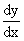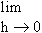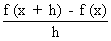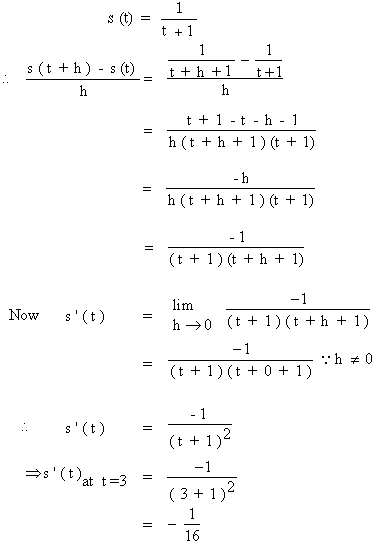Home MonkeyNotes Printable Notes Digital Library Study Guides Study Smart Parents Tips College Planning Test Prep Fun Zone Help / FAQ How to Cite New Title Request

4.5 Interpretation Of Derivative

1. The derivative of a function at a point is the slope of the tangent line at that point. It may be thought of the limiting position of the secant line to a curve y = f (x) at a point is the tangent at the point. Thus the limit of the slope of the secant line through a fixed point on the curve and any other points on the curve that get closer and closer to the fixed point when this limit exists. It is the slope of the tangent line at that fixed point (x, f (x)) on the curve y = f (x).

2. The derivative is the instantaneous velocity of a function representing the position of a particle along a line at time t where y = f (x). The derivative may be thought of as the limit of the average velocities between a fixed time and other times that get near and nearer to this fixed time. When this limit exists, the instantaneous velocity at time t for y = f (t).

3. The derivative is the instantaneous rate of change of a function at a point. It may be thought of as the limit of the average rates of change between a fixed point and other point on the curve that get closer and closer to that fixed point. When this limits exists, it is known as the instantaneous rate of change of the function y = f (x) at the fixed point (x, f (x) ).

Note : When you are asked to findif y = f (x); by first principle of derivatives, use the definition.

i.e.=if it exists.Your browser does not support the IFRAME tag.

Example 5

Find the instantaneous velocity of s (t) =using first principle of derivative.The negative sign indicates that the particle is moving in the negative direction.

Index

4. 1 Derivability At A Point
4. 2 Derivability In An Interval
4. 3 Derivability And Continuity Of A Function At A Point
4. 4 Some Counter Examples
4. 5 Interpretation Of Derivatives
4. 6 Theorems On Derivatives (differentiation Rules)
4. 7 Derivatives Of Standard Functions
4. 8 Derivative Of A Composite Function
4. 9 Differentiation Of Implicit Functions
4.10 Derivative Of An Inverse Function
4.11 Derivatives Of Inverse Trigonometric Functions
4.12 Derivatives Of Exponential & Logarithmic Functions
4.13 Logarithmic Differentiation
4.14 Derivatives Of Functions In Parametric Form
4.15 Higher order Derivatives

Chapter 5